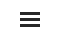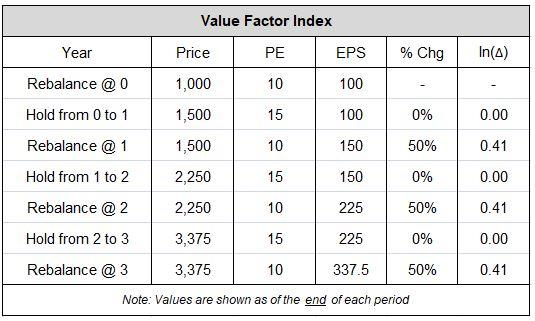# Appendix C: Computing Holding Growth and Rebalancing Growth

By Jesse Livermore+, Chris Meredith, Patrick O’Shaughnessy
May 2018

Sign up to receive a monthly email summary of both our research and the best of what we learned from others.
SUBSCRIBE

To calculate the holding growth and rebalancing growth of a strategy over a given period, we start by separating the period into distinct holding periods and rebalancing events. We then compute the logarithmic change in the fundamental that occurs over each holding period and rebalancing event. We then sum the logarithmic changes together to obtain logarithmic numbers for the overall holding growth and rebalancing growth, respectively. Finally, we scale those numbers up to the overall annualized growth number.

The table below illustrates using data from the example in the piece:After separating out all of the holding periods and rebalancing events, we calculate the logarithmic change in EPS that occurs in each period and event. In the case above, there's no logarithmic change in EPS across the holding periods, therefore the overall holding growth contribution is 0% (=0/(.41*3)). The logarithmic change in EPS that occurs on each rebalancing event is 0.41, therefore the overall rebalancing growth contribution is 100% (=0.41*3/(0.41*3)). The overall annualized growth is 50% per year, so we scale up the rebalancing growth to 100% * 50% per year = 50% per year. The holding growth, of course, is just 0%, so there's no need to scale it.